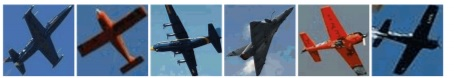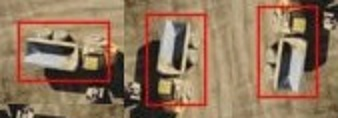2 views

As known, modern most popular CNN (convolutional neural network): VGG/ResNet (FasterRCNN), SSD, Yolo, Yolo v2, DenseBox, DetectNet - are not rotate invariant: Are modern CNN (convolutional neural network) as DetectNet rotate invariant?

Also known, that there are several neural networks with rotate-invariance object detection:

1. Rotation-Invariant Neoperceptron 2006 (PDF): https://www.researchgate.net/publication/224649475_Rotation-Invariant_Neoperceptron

2. Learning rotation invariant convolutional filters for texture classification 2016 (PDF): https://arxiv.org/abs/1604.06720

3. RIFD-CNN: Rotation-Invariant and Fisher Discriminative Convolutional Neural Networks for Object Detection 2016 (PDF): http://www.cv-foundation.org/openaccess/content_cvpr_2016/html/Cheng_RIFD-CNN_Rotation-Invariant_and_CVPR_2016_paper.html

4. Encoded Invariance in Convolutional Neural Networks 2014 (PDF)

5. Rotation-invariant convolutional neural networks for galaxy morphology prediction (PDF): https://arxiv.org/abs/1503.07077

6. Learning Rotation-Invariant Convolutional Neural Networks for Object Detection in VHR Optical Remote Sensing Images 2016: http://ieeexplore.ieee.org/document/7560644/

We know, that in such image-detection competitions as: IMAGE-NET, MSCOCO, PASCAL VOC - used networks ensembles (simultaneously some neural networks). Or networks ensembles in single net such as ResNet (Residual Networks Behave Like Ensembles of Relatively Shallow Networks)

But are used rotation invariant network ensembles in winners like as MSRA, and if not, then why? Why in ensemble the additional rotation-invariant network does not add accuracy to detect certain objects such as aircraft objects - which images is done at a different angles of rotation?

It can be:

• aircraft objects which are photographed from the ground• or ground objects which are photographed from the airWhy rotation-invariant neural networks are not used in winners of the popular object-detection competitions?

by (33.1k points)

The current progress in image recognition is made by changing the approach from a classic feature selection (shallow learning algorithm) to no feature selection (deep learning), due to the mathematical properties of convolutional neural networks. Their ability to capture the same information using a smaller number of parameters was partially caused by their shift-invariance property.

The main reason behind this success was developing faster learning algorithms than more mathematically accurate ones, so less attention is put on developing another property invariant neural nets.